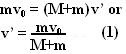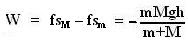## Tuesday, May 20, 2008

### Irodov Problem 1.164As the mass slides down the slope it looses it potential energy and gains speed (kinetic energy). By the time it reaches the plank let its velocity be v0 . Then we have,Now as the disc moves over the plank, it is offered resistance due
the force of friction f between the disc and the mass consequently the disc slows down. The same force of friction f however, pushes the plank forward and accelerates it until finally there is not relative motion between the plank and the disc. This is shown in the figure.

1) From this point the problem can be solved in two different ways, i) using the conservation of momentum and ii) using newton's laws. I shall show both the methods for the sake of interested reader.

Using Conservation of Momentum: Since there is no external force on the (disc + plank) system in the horizontal direction (friction forces are internal to the (disc + plank) system), the momentum of the (disc + plank) system will be conserved along the horizontal direction. When finally both the disc and plank start to move together (there is no relative motion between disc and plank) say that the velocity is v'. Then we have,The total energy will always be conserved and so, initial kinetic energy of the (disc + plank) system + work done by friction (W) = final kinetic energy of the (disc + plank) system . Hence we have,Using Newton's Laws:
Suppose that deceleration of the disc relative to the plank is a and let the acceleration of the plank be a'. Then the deceleration of the disc relative to a stationary frame is given by a-a'. There is only one force acting on both the disc and the plank - the force of friction which acts to eliminate the relative motion between them. Hence we have,Here v'(t) and v(t) are the velocities of the plank and that of the disc relative to the plank respectively. When finally the plank and the mass start to move with the disc, v(t)=0 and so the final velocity of the system isThis the same as we found using conservation of momentum!!!

The total work done by friction is sum of the work done by friction on the plank and the work done by friction on the disc. Let sm be the distance traveled by the disc before it starts moving with the plank as seen in a stationary reference frame. Also let sM be the distance traveled by the plank before the disc stops slipping on the plank. We have,The work done by friction force on the disc will be -fsm, the -ive sign coming because the force of friction and the direction of motion of the disc are in opposite direction. The work done by friction forces on the plank will be fsM. The total work done by friction forces is this,2)
Rather than rigorously proving that the result is independent of reference frame I will give an outline reasoning for the proof. Consider an observer in an arbitrary frame F of reference (could be an accelerated frame) . From the point of view of F both the plank and the disc will experience the same set of pseudo forces and so the magnitude of force of friction (depends only on the relative motion between the disc and the plank) between the plank and the disc will remain the same as seen from the point of view of the stationary frame. Also, the motion of the plank and disc will be always aligned with the direction of the force of friction. Further, the distance traveled (measured along the arbitrary curve that they move along as seen from the accelerated frame) by the disc and the plank before the relative motion between the plank and the disc reduces to zero will also be exactly same as derived in part 1 of the question. In other words the work done by friction will also be the same.

Intuitively thinking suppose that this work done by friction heats up the plank and the disc to a certain temperature as seen in one frame, how can this temperature be different as seen from another reference frame?